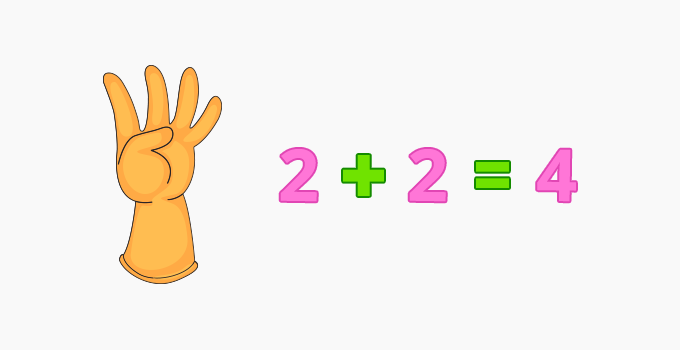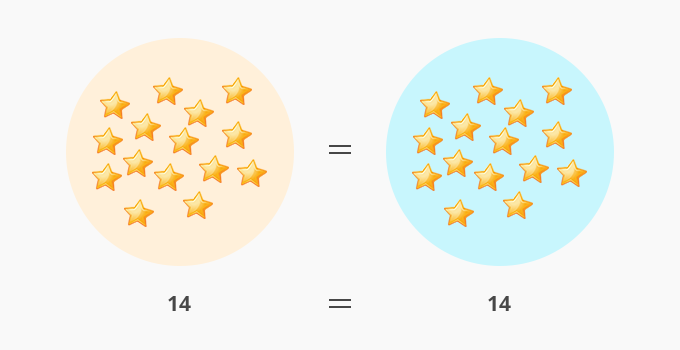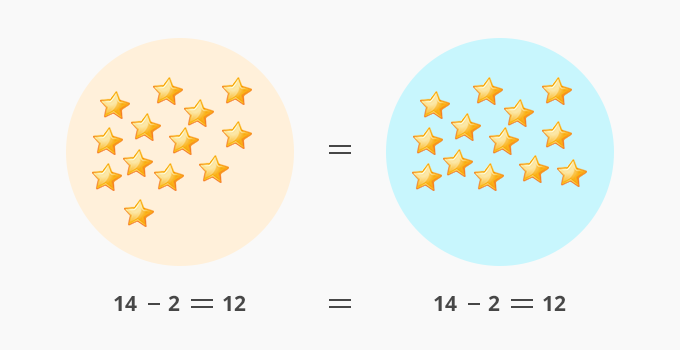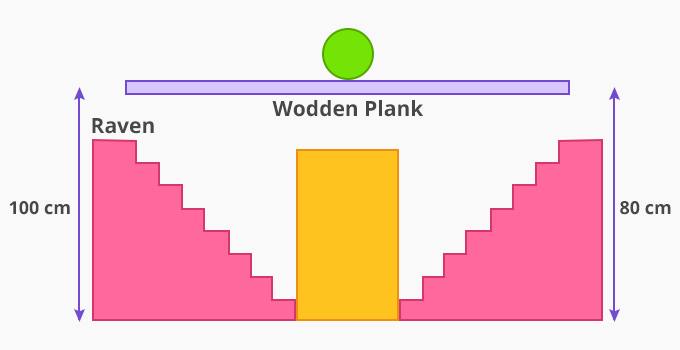Subtraction Property Of Equality - Definition with Examples

The Complete K-5 Math Learning Program Built for Your Child

• 30 Million Kids

Loved by kids and parent worldwide

• 50,000 Schools

Trusted by teachers across schools

• Comprehensive Curriculum

Aligned to Common Core

Subtraction Property of Equality

Equations are expressions having two equal parts on each side of an ‘equal to sign’.Subtraction property of equality

Subtraction property of equality refers to balancing an equation by using the same mathematical operation on both sides.

For instance: We have 2 circles with the same number of stars.Stars in Circle 1 = Stars in Circle 2

= 14 = 14

If we take away 2 stars from Circle 1 then, to balance this equation in both the circles we need to take away 2 stars from the other circle also.So, we can write an equation:

if a = b

then, a - c = b - c

Example 1:John wishes to fly using10 balloons. He has 10 in one hand and 10 in another. As he sets to fly, a bird bursts 3 balloons on one side, making him lose balance. How many balloons should he let go to regain his balance?

Solution:

To solve this question, we have to use the subtraction property of equality.

Initially, the person is holding 10 balloons in both of his hands.

10 = 10

Then, 3 balloons were destroyed by a bird, resulting in a loss of balance.

10 - 3 = 10 ⇒ 7 = 10 [Both sides are not balanced which makes it incorrect]

To balance the other side, John lets go of 3 balloons.

7 = 10 - 3 ⇒ 7 = 7 [Equation is balanced as both sides are equal]

Example 2: Raven and Maddy are playing a game of balancing a ball on a wooden plank. Raven says, “I am going 20 cm down”. To keep the ball balanced on the wooden plank, where should Maddy go?Solution:

To solve this question, we have to use the subtraction property of equality.

Initially, the ball is balanced as Raven and Maddy are at the same height.

70 cm =70 cm

Now, Raven is going 20 cm down. To keep the ball balanced Maddy must also step down by 20 cm  70 - 20 = 70 - 20 ⇒ 50 cm = 50 cm

 Fun Facts Addition and subtraction are inverse operations.

Won Numerous Awards & Honors